# 深度学习与自然语言处理(1)_斯坦福cs224d Lecture 1

http://blog.csdn.net/han_xiaoyang/article/details/51567822
http://blog.csdn.net/longxinchen_ml/article/details/51567960

### 1. 自然语言处理简介

• 语法检查
• 关键词搜索
• 查找同义词

• 从网站，文件或其他来源中提取信息

• 机器翻译（例如：中译英）
• 语意分析（提问者说的意思是什么）
• 指代分析（例如. “他”或“它”在一个特定文件中指的是什么）
• 回答问题（例如.回答“Jeopardy Questions”一种涉及人类社会各个方面的综艺问答）

### 2.词向量

$w^{aardcark}= \begin{bmatrix} 1 \\ 0 \\ 0 \\ \vdots \\ 0 \end{bmatrix} , w^{a}= \begin{bmatrix} 0 \\ 1 \\ 0 \\ \vdots \\ 0 \end{bmatrix} w^{at}= \begin{bmatrix} 0 \\ 0 \\ 1 \\ \vdots \\ 0 \end{bmatrix} \cdots \\ w^{zebra}= \begin{bmatrix} 0 \\ 0 \\ 0 \\ \vdots \\ 1 \end{bmatrix}$

$(w^{hotel})^Tw^{motel}=(w^{hotel})^Tw^{cat}=0$
（注：这里$W^{-1}$$W$的逆矩阵，它们有关系：$W^{-1}*W=1$，注意到hotel和motel是近义词）

### 3.基于SVD的方法

#### 3.2 基于窗口的共现矩阵X

1. I enjoy flying.
2. I like NLP.
3. I like deep learning.
由此产生的计数矩阵如下：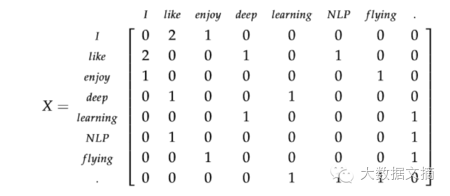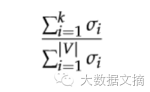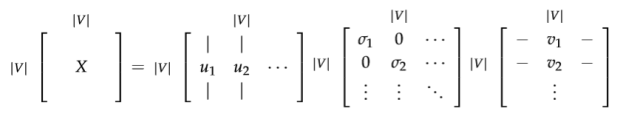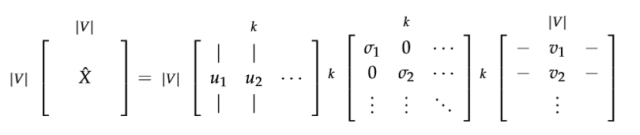• 矩阵的维度会经常变化（新的词语经常会增加，语料库的大小也会随时变化）。
• 矩阵是非常稀疏的，因为大多数词并不同时出现。
• 矩阵的维度通常非常高（$≈10^6×10^6$
• 训练需要$O(n^2)$的复杂度（比如SVD）
• 需要专门对矩阵X进行特殊处理，以应对词组频率的极度不平衡的状况

• 忽视诸如“he”、“the” 、“has”等功能词。
• 应用“倾斜窗口”（ramp window），即:根据文件中词组之间的距离给它们的共现次数增加相应的权重。
• 使用皮尔森的相关性（Pearson correlation），将0记为负数，而不是它原来的数值。

### 4.基于迭代的方法

词语的上下文：



#### 4.1 语言模型（1-gram,2-gram等等）

"The cat jumped over the puddle."（猫 跳 过 水坑）

$P(w_1,w_2,w_3...w_n)$

$P(w_1,w_2,w_3...w_n)=\prod_{i=1}^NP(w_i)$

$P(w_1,w_2,w_3...w_n)=\prod_{i=2}^NP(w_i|w_{i-1})$

#### 4.2 连续词袋模型（CBOM）

• $w_i$:单词表V中的第i个单词
• $v\in R^{n*|V|}$：输入词矩阵
• $v_i$：V的第i列，单词$w_i$的输入向量
• $u\in R^{|V|*n}$：输出词矩阵
• $u_i$：U的第i行，单词$w_i$的输出向量

1. 对于m个词长度的输入上下文，我们产生它们的one-hot向量（$x^{(c-m)},\cdots,x^{(c-1)},x^{(c+1)},\cdots,x^{(c+m)}$）。
2. 我们得到上下文的嵌入词向量（$v_{c-m+1}=Vx^{(c-m+1)},\cdots,$v_{c+m}=Vx^{(c+m)}）
3. 将这些向量取平均$\hat v={v_{c-m}+v_{c-m+1}+\cdots+v_{c+m}\over2m}$
4. 产生一个得分向量 $z=U\hat v$
5. 将得分向量转换成概率分布形式$\hat y=softmax(z)$
6. 我们希望我们产生的概率分布 ,与真实概率分布$\hat y$相匹配。而$y$刚好也就是我们期望的真实词语的one-hot向量。$H(\hat y,y)=-\sum_{j=1}^{|V|}y_jlog(\hat y_j)$

$H(\hat y,y)=-y_ilog(\hat y_i)$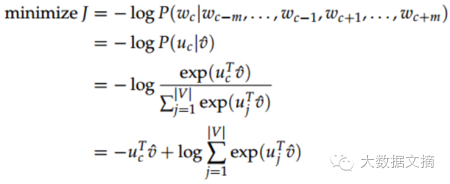#### 4.3 Skip-Gram 模型

Skip-Gram 模型中的各个记号：

• $w_i$:单词表V中的第i个单词
• $v\in R^{n*|V|}$：输入词矩阵
• $v_i$：V的第i列，单词$w_i$的输入向量
• $u\in R^{|V|*n}$：输出词矩阵
• $u_i$：U的第i行，单词$w_i$的输出向量

1. 生成one-hot输入向量x。
2. 得到上下文的嵌入词向量$v_c=Vx$
3. 因为这里不需要取平均值的操作，所以直接是$\hat v=v_c$
4. 通过$u=Uv_c$产生2m个得分向量$u_{c-m},\cdots,u_{c-1},u_{c+1},\cdots,u_{(c+m)}$
5. 将得分向量转换成概率分布形式$y=softmax(u)$
6. 我们希望我们产生的概率分布与真实概率分布$y^{c-m},\cdots,y^{c-1},,y^{c+1}\cdots,y^{c+m}$ 相匹配，也就是我们真实输出结果的one-hot向量。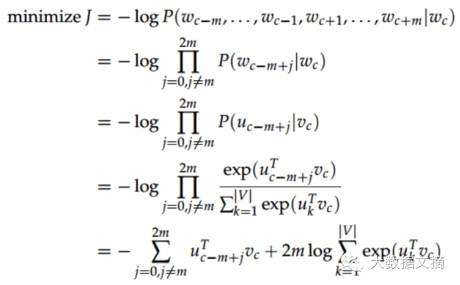#### 4.4 负面抽样（Negative Sampling）

• 目标函数
• 梯度
• 更新规则

Mikolov ET AL.在他的《Distributed Representations of Words and Phrases and their Compositionality》中提出了负面抽样。虽然负面抽样是基于Skip-Gram 模型，它实际上是对一个不同的目标函数进行最优化。考虑一个“词-上下文”对（w,c），令P(D = 1|w, c)为(w, c)来自于语料库的概率。相应的，P(D = 0|w, c) 则是不来自于语料库的概率。我们首先对P(D = 1|w, c)用sigmoid函数建模：
$p(D=1|w,c,\theta)= {1\over{1+e^{(-v_c^Tv_w)}}}$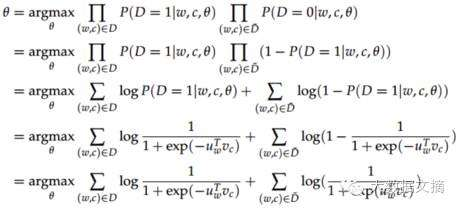$log\sigma(u_(c-m+j)^T.v_c)+\sum_{k=1}^Klog\sigma(-\widetilde u_k^T.v_c)$

$is:0.9^{3/4}=0.92\\ constitution:0.09^{3/4}=0.16 \\ bombastic:0.01^{3/4}=0.032$

06-192万+

#### 深度学习与自然语言处理(3)_斯坦福cs224d Lecture 306-122万+

#### 深度学习与自然语言处理(2)_斯坦福cs224d Lecture 2

06-042133

#### 自然语言处理中传统词向量表示VS深度学习语言模型（一）

07-30334

#### Stanford 深度自然语言处理 学习笔记（一）

04-02657

#### 斯坦福大学-自然语言处理与深度学习（CS224n） 笔记 第一课 介绍

06-262万+

#### 深度学习与自然语言处理(4)_斯坦福cs224d 大作业测验1与解答

10-022395

#### 斯坦福CS224N_自然语言处理NLP深度学习DL课程笔记（一）©️2020 CSDN 皮肤主题: 猿与汪的秘密 设计师: 上身试试点击重新获取扫码支付1.余额是钱包充值的虚拟货币，按照1:1的比例进行支付金额的抵扣。
2.余额无法直接购买下载，可以购买VIP、C币套餐、付费专栏及课程。余额充值